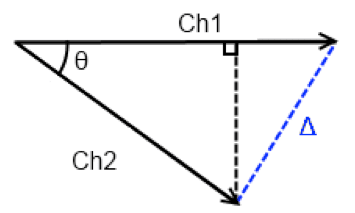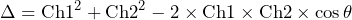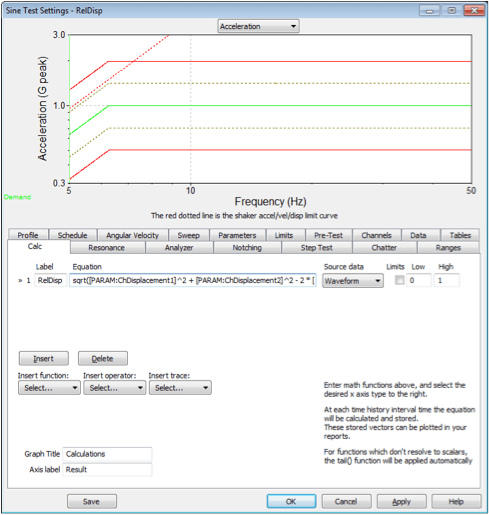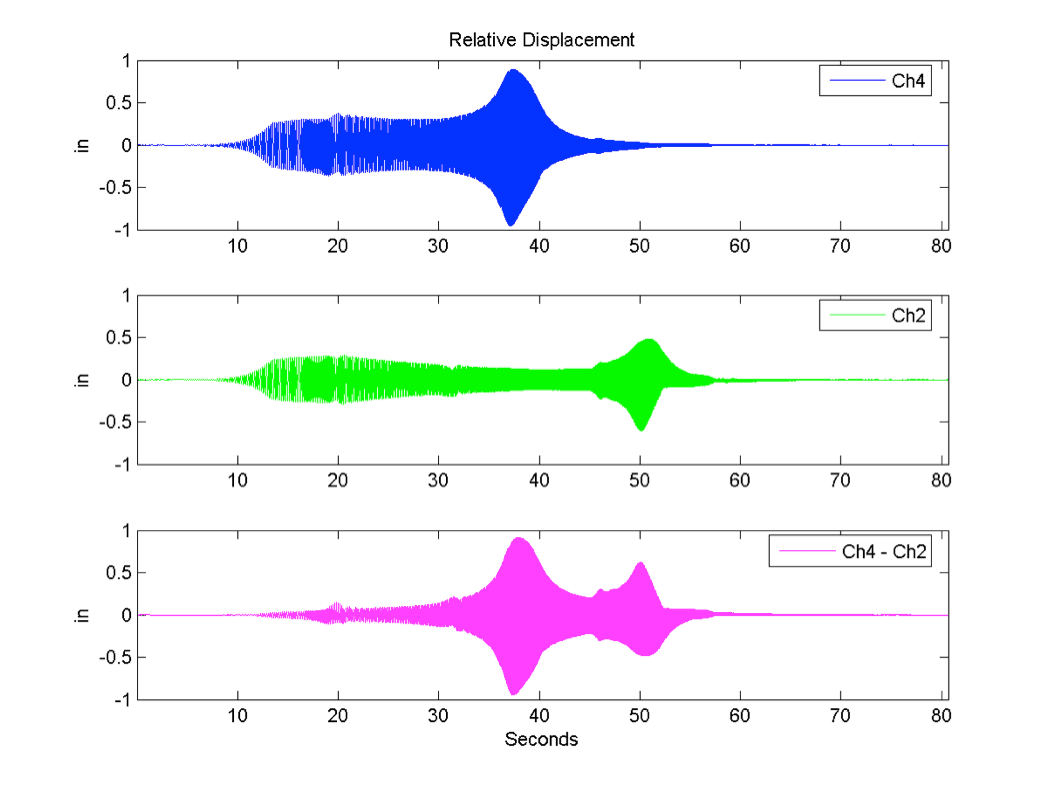# Computing Relative Displacement Between Channels

## Abstract

Two methods of calculating the relative displacement between two channels are described.

### Question

Vibration Research often receives questions about how to compute the relative displacement between two channels. There are two ways this can be accomplished; the trade-off between the two is accuracy and ease of use.

Computing relative displacement is not just a matter of subtracting channel 2 from channel 1. This method only works for a pure sine tone with the channels perfectly in phase.

For example, channel 1 and channel 2 are both experiencing a sine tone with an amplitude of 1″. If the two channels are perfectly in phase, the relative displacement between them will be 0. If they are 180 degrees out of phase, their relative displacement will be 2″.

#### Calculator Method

During a sine test, the engineer can filter out the harmonics and calculate the relative displacement by assuming a pure sine tone. They need to take the phase into account to do so.

Start by plotting the signal for channel 1 and channel 2 as vectors with the phase between the two channels as θ.Ch1 and Ch2 as vectors with a phase of θ.

The following equation calculates the relative displacement between Ch1 and Ch2 (Δ).

(1)Equation 1

Calculator Method in VibrationVIEW

Using Equation 1 in VibrationVIEW requires the Calculator feature (VR9606). Navigate to the Calculator tab in the Sine Test Settings dialog, and select the Insert button to add a new line. Enter the label as “RelDisp” and enter the following equation. The source data should be “Waveform.”

sqrt(([PARAM:ChDisplacement1]/2)^2 + ([PARAM:ChDisplacement2]/2)^2 – 2 * ([PARAM:chDisplacement1]/2) * ([PARAM:ChDisplacement2]/2) * cos(([PARAM:ChPhase1] – [PARAM:ChPhase2])*pi/180) )Equation field in Calc tab of the Sine Test Settings.

The [PARAM:ChDisplacement1] is divided by 2 because the PARAM value is peak to peak. The equation also requires the amplitude.

When the test is running, this calculator trace will continually calculate the relative displacement between Ch1 and Ch2. The results can be graphed versus time (waveform) or frequency. Select the Edit Graph button and select the Calculator vs Freq. radio button. Then, select the RelDisp math trace under Graph Traces to the right.An example of the results is below. In this case, the maximum relative displacement between channels 2 and 4 is 0.889″ and occurs at 11.53Hz.Example results using the calculator method to calculate relative displacement.

This method only works for a sine test and calculates the relative displacement at the excitation frequency. It does not take other harmonics into account.

#### Post-processing Method

To calculate the relative displacement across a broad frequency range, the engineer must record the entire waveform. Then, they can use a third-party tool such as MatLab or Octave to calculate the relative displacement.

If the engineer uses a displacement transducer to record the data, then they can apply a simple point-by-point subtraction to calculate the relative displacement. However, recorded data typically comes from accelerometers. In this case, they will need to convert the data from acceleration to displacement.

One way to convert from acceleration to displacement is to double-integrate using the cumulative sum. This method is prone to random walk so all that is visible is very low-frequency random noise. A better way to convert is to apply a 2nd order low-pass-filter with a corner frequency below the minimum frequency of interest. This method requires the Signal Processing Toolkit for MatLab.

Post-processing Method Example

The above script was run on a recording from the previous calculator method example. The results are below.There are some differences in the output. The calculator method only shows the relative displacement. The post-processing method also shows the two channels in relationship to each other. Additionally, the post-processing method takes all the frequencies into account when calculating the relative displacement.

The graph above shows the displacement waveform for channels 4 and 2 and the relative displacement between the two. It may not be clear from the graph above, but the maximum and minimum values do not occur at the same time. The maximum value of 0.91″ occurs at 37.9 seconds and the minimum value of -0.94″ occurs at 37.5 seconds.

maxRelDisp = 0.9134 maxTime = 37.9397 maxFreq = 11.4167
minRelDisp = -0.9440 minTime = 37.4541 minFreq = 11.2219

For this test, the COLA was configured to output DC voltage proportional to the sine test frequency and looped back to channel 3. Channel 3 was configured as 1000mv/V with a DC signal. This configuration allowed us to see that the frequency at the time of the maximum relative displacement was 11.4 Hz. The minimum relative displacement was 11.2 Hz.

In this case, the difference is rather insignificant and is only about 5.5 cycles but it shows that the maximum and minimum relative displacement does not have to occur at the same time.

This example uses a sine test, but this method works with a random test as well.